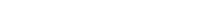# Math riddles Level 43 Answer with Hints and solutionsMath riddles game level 43 Answer with solution android game developed by Black games. Scroll Below to find details.

Math Riddles tests your IQ with mathematical puzzles. Challenge yourself with different levels of math puzzles and stretch the limits of your intelligence. Every IQ game is prepared with an approach of an IQ test. Improve your mathematics, challenge your brain with this game. There are many hard levels which you may not have solved then you can take help from here.

Math app level 43:

7,3=32

6.10=77

8,2=27

5,7=?Solution:

(7+1)*(3+1)=32

(6+1)(10+1)=77

(8+1)(2+1)=27

(5+1)(7+1)=48

If there is any Doubt/discrepancy then please let me know in comments or you are unable to understand solution then I will surely help you.

### 5 thoughts on “Math Riddles App Level 43 Solution Android”

•August 18, 2019 at 11:13 pm

There is also (a*b)+11

•July 31, 2019 at 11:55 pm

It’s funny to see how everyone’s brain works differently. I solved it, but did a different formula. a+(a×b)+b+1

•May 26, 2019 at 6:08 am

ther is no logic in this one ?

•February 13, 2019 at 2:20 pm

There is an other solution to:

(7*3)+11=32
(6*10)+17=77
(8*2)+11=27
(5*7)+17=52

•